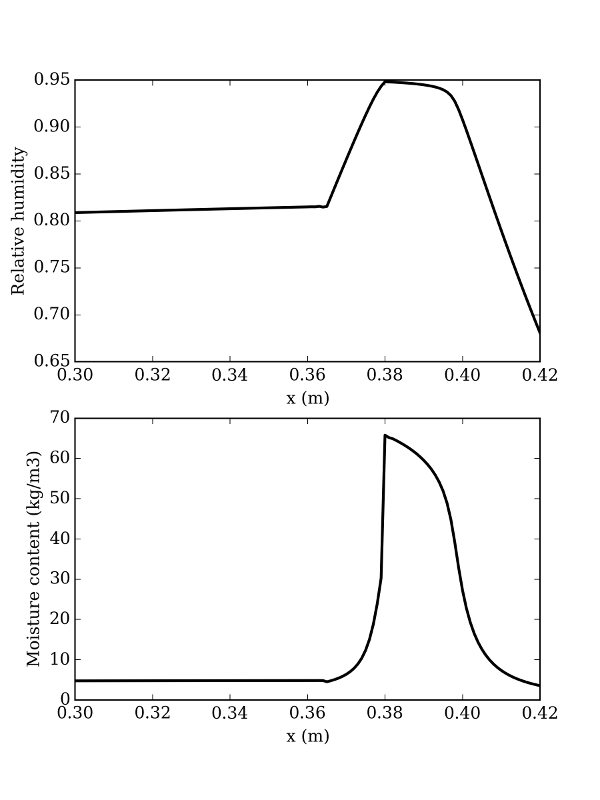This script simulates the fifth exercise of the Hamstad benchmark package: multi-layered wall with capillary active inside insulation

Since hamopy is not really speed-optimised, this may take a few minutes to compute.

## Script

``````"""
5th exercise of the Hamstad benchmark package

Capillary active inside insulation
"""

import numpy  as np
import matplotlib.pylab as plt

# All things necessary to run the simulation
from hamopy import ham_library as ham
from hamopy.classes import Mesh, Boundary, Time
from hamopy.algorithm import calcul
from hamopy.postpro import distribution

# Choice of materials and geometry
from hamopy.materials.hamstad import BM5_brick, BM5_mortar, BM5_insulation

mesh = Mesh(**{"materials"    : [BM5_brick, BM5_mortar, BM5_insulation],
"sizes"        : [0.365, 0.015, 0.040],
"nbr_elements" : [100, 20, 20] })

# Boundary conditions
clim1 = Boundary('Fourier',**{"T"   : 273.15,
"HR"  : 0.8,
"h_t" : 25.,
"h_m" : 1.8382e-7 })

clim2 = Boundary('Fourier',**{"T"   : 293.15,
"HR"  : 0.6,
"h_t" : 8.,
"h_m" : 5.8823e-8 })

clim = [clim1, clim2]

# Initial conditions
init = {'T'  : 298.15,
'HR' : 0.6}

# Time step size
time = Time('variable',**{"delta_t"  : 900,
"t_max"    : 12960000,
"iter_max" : 12,
"delta_min": 1e-3,
"delta_max": 900 } )

# Calculation
result = calcul(mesh, clim, init, time)

# Post processing
t_plot = 12960000
x_plot = np.linspace(0, 0.42, 421)
Temperature = distribution(result, 'T', x_plot, t_plot)
Humidity    = distribution(result, 'HR', x_plot, t_plot)
Moisture    = np.zeros(np.shape(Temperature))

for i in range(len(mesh.materials)):
xmin = sum(mesh.sizes[0:i])
xmax = sum(mesh.sizes[0:i+1])
mask = ((x_plot >= xmin) & (x_plot <= xmax))

# Plotting results
fig, ax = plt.subplots(2, 1)
ax.plot(x_plot[300:], Humidity[300:], 'k-', linewidth=2)
ax.set_xlabel('x (m)')
ax.set_ylabel('Relative humidity')
ax.plot(x_plot[300:], Moisture[300:], 'k-', linewidth=2)
ax.set_xlabel('x (m)')
ax.set_ylabel('Moisture content (kg/m3)')
plt.show()
``````

## Results